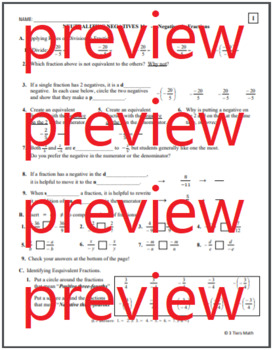# 7.NS.2 Negatives in Fractions - Move those Negatives to the Numerator!Subject
Resource Type
File Type

PDF

(445 KB|11 pages)
Standards
Also included in:
1. Start students off confidently with the 3 Tiers original Stack, Add or Subtract, Leader of the pack adding model. Then work through subtraction, absolute value, multiplication, and division, engaging fractions decimals and word problems throughout. Includes bonus set of flashcard / notes hybrid th
\$13.00
\$9.00
Save \$4.00
• Product Description
• StandardsNEW

When students struggle to correctly assess common denominators, mixed numbers, and improper fractions with the 4 operations, throwing negatives in the mix is devastating. If your students have trouble interpreting the various placement of negatives and subtraction signs on fractions, this lesson is for you!

Students are guided to explore the meaning of a fraction when negatives are placed in various locations and combinations, leading to recognizing equivalent fractions. They are specifically prompted to write negative fractions with the negative in the numerator as an aid to completing the 4 operations.

Then, students engage a hands on sorting activity to remind them of all the things to keep track of when working with fractions. Finally, they apply all they’ve learned to practice solving various operations with negative and positive fractions.

About the 3 TIERS MATH model:

We have analyzed and targeted the known struggles for this topic by scaffolding the lesson in 3 differentiated versions: Independent, Hints, and Guided. Word banks, answer keys, sentence starters, templates, hints, problem chunking and other strategies are used to provide a means for less confident students access the same curriculum and rigor, allowing all students to engage in one common learning experience.

This lesson is designed for students to work through in pairs or groups to discover the big ideas through guided inquiry. While some direct instruction is required, the structure empower students to be independent learners, where your role transitions to more of a mentor than teacher. It is the eleventh lesson in the Neutralizing Negatives Unit for a 7th grade Common Core math class. There is a different lesson that is geared toward a 7th grade Accelerated Math class (not included).

Solve real-world and mathematical problems involving the four operations with rational numbers.
Apply and extend previous understandings of multiplication and division and of fractions to multiply and divide rational numbers.
Apply and extend previous understandings of addition and subtraction to add and subtract rational numbers; represent addition and subtraction on a horizontal or vertical number line diagram.
Total Pages
11 pages
Included
Teaching Duration
50 minutes
Report this Resource to TpT
Reported resources will be reviewed by our team. Report this resource to let us know if this resource violates TpT’s content guidelines.